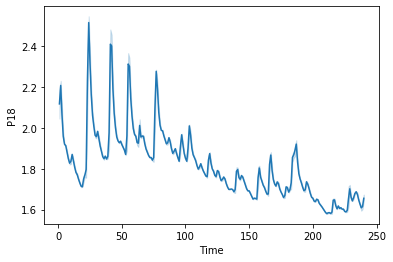# Setting up a conv1d (updated)

Hi all,

Just (UPTADED) got rid of one error but now I got a new one:), playing grown-up lego is hard.

I am just starting my deep learning journey, and I am trying to fit an ( i believe 1d problem ) to a CNN,

I have a dataset which I already manipulate a little bit, so the signals are in equal length and on a correct format,

The dataset has 64 observations with 1024 attributes (timestamps). I tried to set up a convolutional 1d network, but I am getting a strange error:

RuntimeError: size mismatch, m1: [32 x 64], m2: [2048 x 128] at C:/w/1/s/tmp_conda_3.7_100118/conda/conda-bld/pytorch_1579082551706/work/aten/src\THC/generic/THCTensorMathBlas.cu:290

So, the way I thought about the problem was, if I have a 32 size batch of 1024 attributes and is one dimensional I would set the first conv layer as nn.Conv1d(1024, 32, 1, 4) then second conv layer as self.conv2 = nn.Conv1d(32, 64, 1 ,4). I understand the kernel has to be one ( I’m i right?) … Please see my code below:

``````class MulticlassClassification(nn.Module):
def __init__(self):
super(MulticlassClassification, self).__init__()
self.conv1 = nn.Conv1d(1024, 32, 1, 4)
self.conv2 = nn.Conv1d(32, 64, 1 ,4)
self.dropout1 = nn.Dropout2d(0.25)
self.dropout2 = nn.Dropout2d(0.5)
self.fc1 = nn.Linear(2048, 128) ## Im I getting this part right ( 32 * 64)
self.fc2 = nn.Linear(128, 3)

def forward(self, x):
x = x.unsqueeze(2) # to take care of the shape
x = self.conv1(x)
x = F.relu(x)
x = self.conv2(x)
x = F.relu(x)
x = F.max_pool2d(x, 2)
x = self.dropout1(x)
x = torch.flatten(x, 1)
x = self.fc1(x)
x = F.relu(x)
x = self.dropout2(x)
x = self.fc2(x)
output = F.log_softmax(x, dim=1)
return output
``````

Any help will be much appreciated. Another quick question, I am sensibly approaching the problem? Perhaps should I transform the signals as an image or is an overkill 28x28?

Many thanksI’m not sure about the model architecture.
Based on your description you are dealing with inputs of `[batch_size, 1024]` and are unsqueezing this input tensor to create a fake temporal dimension to `[batch_size, 1024, 1]`.
If that’s the case, the `nn.Conv1d` with a kernel size of 1 will be a linear layer, as each kernel will connect to all input channels and create a single output value. These output activations will then to concatenated to the shape `[batch_size, nb_filters, 1]`, which should be equal to the usage of `nn.Linear`.

That being said, the raised error comes from a shape mismatch in the first linear layer, as your input activation contains 64 features (number of filters in `self.conv2`), while `self.fc1` expects 2048 input features.
Change the `in_features` to 64 for `self.fc1` and it should work.

Hi Patrick,

Thank you so much. I actually manage to make work. I will post here some intructions on how i did to help future users.

Ian

Hi Patrick,

The data is a time series of 1024 in len. What you mean about fake temporal dimension ( the data has about 4:26 minutes because the points are signals in 4hz) , i actually change now the kernel size and padding a bit, to see how the output behaves. I change now the kernels to size of 3 with padding of one. I know understood the kernel one and linear, because I had a simpler vanilla model and the accuracy was equal.

Thanks for the time you took in explaining very clear what is happening on my code.

Ian